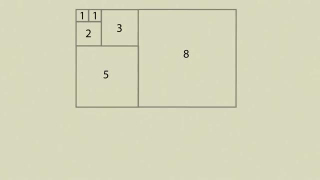2:40
Posted 23 January 2014
Magic of the Fibonacci numbers
(part two)

# Magic of the Fibonacci numbers(part two)

Posted on 23 January 2014
Description
One day, professor Arthur T. Benjamin decided that mathematics is not that boring as almost everyone thinks. Having decided so, he wrote a book called "The Joy of Mathematics". And then he also started giving speeches to entertain the audience. In this video, he is going to share his thoughts about the Fibonacci numbers.
рубрики:
Fibonacci numbers
Recommended words
and so on -
application -
an area -
base -
to calculate -
calculation -
check out -
clearly -
correctly -
to discover -
to divide -
to draw -
equal -
an equation -
to fascinate -
figure out -
to generate -
height -
inside -
on the one hand -
on the other hand -
a pattern -
a ratio -
a rectangle -
satisfying -
simple -
solve for -
square -
squared -
to summarize -
to understand -